# Normal curvature

of a regular surface

A quantity that characterizes the deviation of the surface at a pointin the directionfrom its tangent plane and is the same in absolute value as the curvature of the corresponding normal section. The normal curvature in the directioniswhereis the curvature of the normal section in the direction,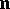is the unit principal normal vector of the normal section andis the unit normal vector to the surface. The normal curvature of a surface in a given direction is the same as that of the osculating paraboloid in this direction. The normal curvature of a surface parametrized byandcan be expressed in terms of the values of the first and second fundamental forms of the surface (cf. Fundamental forms of a surface) computed for the values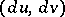corresponding to the directionby the formula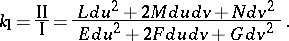The curvature of a regular curve lying on a surface is connected with the normal curvature of the surface in the direction of the unit tangentto the curve and with the geodesic curvature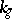of the curve by the relation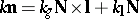(see also Meusnier theorem). By means of the normal curvature one can construct the Dupin indicatrix, the Gaussian curvature and the mean curvature of the surface, as well as many other concepts of the local geometry of the surface.